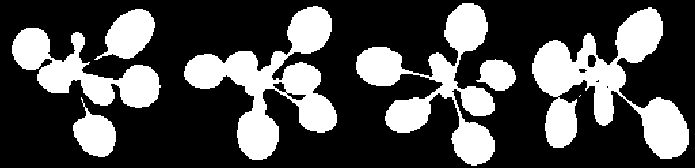Semantic segmentation refers to the task of assigning a label to each pixel in the image. This can be used, for example, to segment organs from a plant by using the labelling as a segmentation mask. Although it may be overkill compared to standard thresholding indices, it can also be used to segment plants from the background as in this example, which is the basis of the vegetation segmentation tool.DPP provides the option to use fully convolutional networks in order to perform the semantic segmentation task. By default, binary segmentations are performed (pixels are assigned a number between 1 and 0, which can be rounded or thresholded to get a mask); segmentations with multiple classes can also be performed with some small changes to the settings.

## Example

Here is a simple fully convolutional network for binary segmentation of images.

``````import deepplantphenomics as dpp

model = dpp.SemanticSegmentationModel(debug=True, save_checkpoints=False, report_rate=20)

# 3 channels for colour, 1 channel for greyscale
channels = 3

# Setup and hyper-parameters
model.set_batch_size(4)
model.set_image_dimensions(256, 256, channels)
model.set_resize_images(True)

model.set_test_split(0.2)
model.set_validation_split(0.0)
model.set_learning_rate(0.0001)
model.set_weight_initializer('normal')
model.set_maximum_training_epochs(100)

# Augmentation options
model.set_augmentation_brightness_and_contrast(True)

# Define a model architecture

model.add_convolutional_layer(filter_dimension=[3, 3, channels, 16], stride_length=1, activation_function='relu')
model.add_convolutional_layer(filter_dimension=[3, 3, 16, 32], stride_length=1, activation_function='relu')
model.add_convolutional_layer(filter_dimension=[5, 5, 32, 32], stride_length=1, activation_function='relu')

# Begin training the segmentation model
model.begin_training()
``````

The crucial part here is that you create a `SemanticSegmentationModel` specifically. This will automatically make the output layer into a convolutional layer, which is what you need to output masks instead of scalar values. Also important is the use of `model.load_dataset_from_directory_with_segmentation_masks()`, which loads binary images of ground-truth segmentations as the labels, instead of something like numbers from a csv file. These ground-truth images are `.png` files, with the value 0 in every channel for negative pixels, and the value 255 in every channel for positive pixels.

The only augmentation strategy currently compatible with fully convolutional networks is the brightness and contrast option.

#### Changes for Multi-class Segmentation

The above example can easily be changed to build a model for segmenting images into multiple classes. The only extra setting needed is to add:

``````model.set_num_segmentation_classes(3)
``````

This not only sets the number of classes, but it sets the correct loss function; binary segmentation uses sigmoid cross entropy for its loss, while multi-class segmentation uses softmax cross entropy. Using `set_loss_function` to set the wrong loss function will result in an error during training.

The ground-truth masks are expected to be different as well, although they are loaded the same way. Instead of having pixel values of 0 and 255, they should have integer values mapping to the corresponding class for that pixel; the background and two different objects could be labeled as 0, 1, and 2 respectively.

## Generating and Applying the Segmentation Mask

The fully connected output is a one-channel greyscale image for binary segmentation; it must be rounded or thresholded to get a binary mask. The vegetation segmentation tool does this using the Otsu thresholding method in OpenCV.

Multi-class segmentation will instead produce an image with as many channels as classes. The channel value that is highest corresponds to the predicted class for that pixel, so a one-channel mask can be generated by applying an argmax function to each pixel.

Since the size of the output layer cannot change, the masks are always output in the same dimensions. This means that the mask must be resized to the same width and height as the original image before being applied. See the vegetation segmentation tool for an example of how to do this.